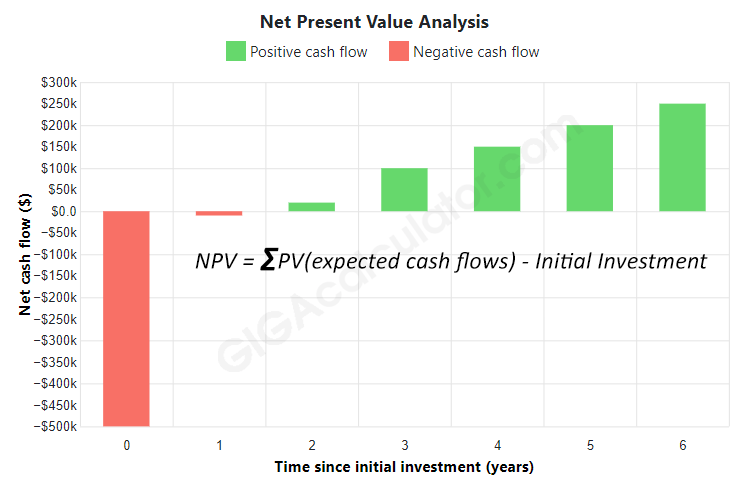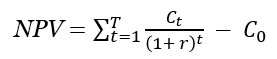# NPV Calculator

Use this online calculator to easily calculate the NPV (Net Present Value) of an investment based on the initial investment, discount rate and investment term. Also calculates Internal Rate of Return (IRR), gross return and net cash flow.

\$
%

Cash flow

\$
\$
\$

### Calculation results

Net Present Value \$77,453.84
Internal Rate of Return 10.076%
Gross Return 34%
Net Cash Flow \$170,000

#### Net Present Value Analysis

Positive cash flow   Negative cash flow
Share calculator:

Embed this tool:
get code

## Using the NPV calculator

Our online Net Present Value calculator is a versatile tool that helps you:

• calculate the Net Present Value (NPV) of an investment
• calculate gross return, Internal Rate of Return IRR and net cash flow

Start by entering the initial investment and the period of the investment, then enter the discount rate, which is usually the weighted average cost of capital (WACC), after tax, but some people prefer to use higher discount rates to adjust for risk, opportunity cost and other factors. This is entirely up to you. Finally, enter the net cash flow for each year or other period (a maximum of 25 periods are allowed). Make sure you enter the free cash flow and not a cash flow after interest, which will result in double-counting the time value of money.

Our NPV calculator will output: the Net Present Value, IRR, gross return, and the net cash flow over the entire period.

## What is Net Present Value?

The definition of net present value (NPV), also known as net present worth (NPW) is the net value of an expected income stream at the present moment, relative to its prospective value in the future meaning it is discounted at a given rate. It is simply a subtraction of the present values of cash outflows (initial cost included) from the present values of cash flows over time, discounted by a rate that reflects the time value of money. The textbooks definition is that the net present value is the sum (Σ) of the present value of the expected cash flows (positive or negative) minus the initial investment.Another way to understand what is meant by "present value" is to consider a situation in which one considers a business investment of \$500,000 expected to bring a cash flow of \$50,000 in one year time or a return on capital of 10%. If the cost of capital is 11% per year then the present value of that \$50,000 income stream is in fact negative (-\$4,504.50 to be exact) meaning that the return does not justify the investment. However, if the cost of capital can be reduced to 5% then the present net worth of this same cash flow would become 23,810 USD signalling a more efficient use of capital so it would be worthwhile to undertake the business venture.

### How to choose the discount rate in NPV analysis?

To estimate the present value we need to set a discount rate. It is inherently company-specific as it relates to how the company is funding its operations. Using WACC is fine in the case of borrowed capital whereas if it is calculated from the point of view of investors and shareholders it can be chosen so it reflects the rate of return they expect. For example, if shareholders expect a 10% return then this is the discount rate to use when calculating NPV for that business.

After the discount rate is chosen, one can proceed to estimate the present values of all future cash flows by using the NPV formula. Then just subtract the initial investment from the sum of these PVs to get the present value of the given future income stream.

## NPV formula

If you wonder how to calculate the Net Present Value (NPV) by yourself or using an Excel spreadsheet, all you need is the formula:where r is the discount rate and t is the number of cash flow periods, C0 is the initial investment while Ct is the return during period t. For example, with a period of 10 years, an initial investment of \$1,000,000 and a discount rate of 8% (average return from an investment of comparable risk), t is 10, C0 is \$1,000,000 and r is 0.08.

## A practical example

Let us see an example of using the Net Present Value calculation to assess the profitability of purchasing a house. Let us say the house costs \$500,000 and it is expected that it could be sold for \$700,000 in 3 years. Maintenance and taxes cost \$10,000 a year. At the same time a less risky investment is a T-Bond which has a yield of 5% per year, meaning that this will be our discount rate. Plugging in the numbers into the Net Present Value calculator we see that the resulting NPV is \$77,454 which is not a bad compensation for the increased risk. We can also compare the IRR which is 10% which is double the T-Bond yield of 5%. Of course, if the risk is more than double that of the safer option, the investment might not be wise, after all.

Note that only the initial investment is an exact number in the above calculation. All other values are estimates and expectations. If they are off by a certain amount, for example if the sale price at the end is only \$650,000 and if the maintenance turns out to be twice as expensive, the investment may yield close to zero discounted return.

## Applications, caveats, and alternatives to net present value

Due to its simplicity, the net present value financial metric is often used to determine whether a project is worth undertaking or an investment worth making as it shows whether it will result in a net profit or loss, with positive NPV meaning that there is profit to be made and negative NPV means losses are to be expected. Usually a company or individual cannot pursue every positive return project, but NPV is still useful as a tool in discounted cash flow (DCF) analysis used to compare different prospective investments.

As with any metric, NPV is only as accurate as long as the assumptions are met and the estimates that go in are well-researched. Substantial errors in the output can result from bad input. It is always wise to allow for some unforeseen expenditures to get off the ground or during its duration.

An alternative to net present value is using the payback period, which measures how long it will take for the original investment to be fully repaid, but this method should not be used for longer-term investments as it does not account for the time value of money. Also, it does not reflect earnings past this period and can't account for sharp movements in the cash flow.

Since NPV does not provide an overall net gains/losses picture, it is often used alongside tools such as IRR.

## Financial caution

This is a simple online NPV calculator which is a good starting point in estimating the Net Present Value for any investment, but is by no means the end of such a process. You should always consult a qualified professional when making important financial decisions and long-term agreements, such as long-term bank deposits. Use the information provided by the calculator critically and at your own risk.

#### Cite this calculator & page

If you'd like to cite this online calculator resource and information as provided on the page, you can use the following citation:
Georgiev G.Z., "NPV Calculator", [online] Available at: https://www.gigacalculator.com/calculators/npv-calculator.php URL [Accessed Date: 06 Jul, 2022].